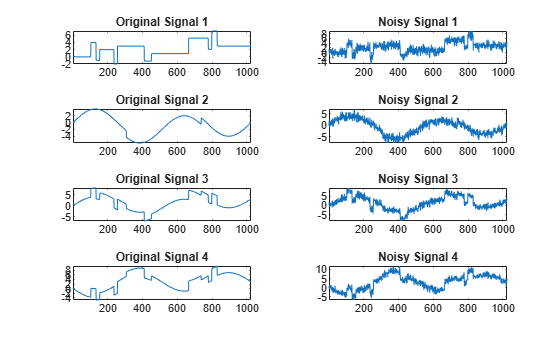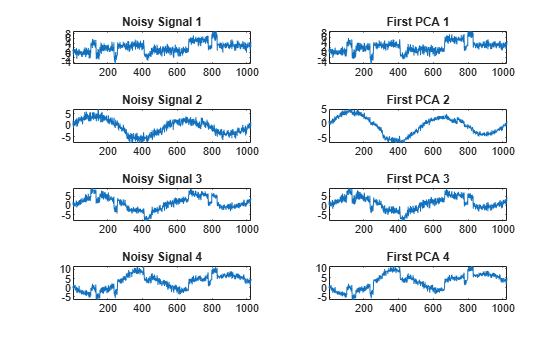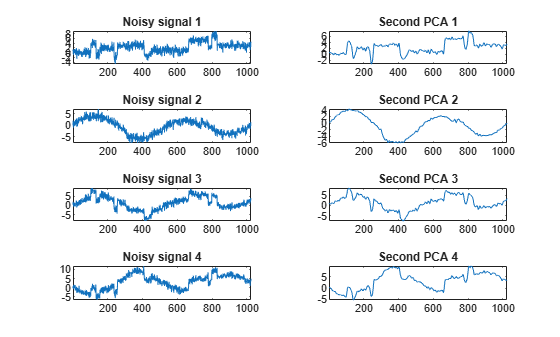# wmspca

Multiscale principal component analysis

## Syntax

``[xsim,qual,npc_out,decsim,pca_params] = wmspca(x,level,wname,npc_in)``
``[___] = wmspca(x,level,wname,'mode',extmode,npc_in)``
``[___] = wmspca(dec,npc_in)``

## Description

example

````[xsim,qual,npc_out,decsim,pca_params] = wmspca(x,level,wname,npc_in)` returns a simplified version `xsim` of the input matrix `x` obtained from the wavelet-based multiscale principal component analysis (PCA). The wavelet decomposition is performed using the decomposition level `level` and the wavelet `wname`.```
````[___] = wmspca(x,level,wname,'mode',extmode,npc_in)` uses the specified discrete wavelet transform (DWT) extension mode `extmode`.```
````[___] = wmspca(dec,npc_in)` uses the wavelet decomposition structure `dec`. `dec` is expected to be the output of `mdwtdec`.```

## Examples

collapse all

Use wavelet multiscale principal component analysis to denoise a multivariate signal.

Load the dataset consisting of four signals of length 1024. Plot the original signals and the signals with additive noise.

```load ex4mwden for i = 0:3 subplot(4,2,2*i+1) plot(x_orig(:,i+1)) axis tight title(['Original signal ',num2str(i+1)]) subplot(4,2,2*i+2) plot(x(:,i+1)) axis tight title(['Noisy signal ',num2str(i+1)]) end```Perform the first multiscale wavelet PCA using the Daubechies least-asymmetric wavelet with four vanishing moments, `sym4`. Obtain the multiresolution decomposition down to level 5. Use the heuristic rule to decide how many principal components to retain.

```level = 5; wname = 'sym4'; npc = 'heur'; [x_sim,qual,npcA] = wmspca(x,level,wname,npc);```

Plot the result and examine the quality of the approximation.

```for i = 0:3 subplot(4,2,2*i+1) plot(x(:,i+1)) axis tight title(['Noisy signal ',num2str(i+1)]) subplot(4,2,2*i+2) plot(x_sim(:,i+1)) axis tight title(['First PCA ',num2str(i+1)]) end````qual`
```qual = 1×4 97.4372 94.5520 97.7362 99.5219 ```

The quality results are all close to 100%. The `npc` vector gives the number of principal components retained at each level.

Suppress the noise by removing the principal components at levels 1-3. Perform the multiscale PCA again.

```npcA(1:3) = zeros(1,3); [x_sim,qual,npcB] = wmspca(x,level,wname,npcA);```

Plot the result.

```for i = 0:3 subplot(4,2,2*i+1) plot(x(:,i+1)) axis tight title(['Noisy signal ',num2str(i+1)]) subplot(4,2,2*i+2) plot(x_sim(:,i+1)) axis tight title(['Second PCA ',num2str(i+1)]) end```## Input Arguments

collapse all

Multisignal, specified as a real-valued matrix. The matrix `x` contains P signals of length N stored column-wise (N > P).

Data Types: `double`

Level of decomposition, specified as a positive integer. `wmspca` does not enforce a maximum level restriction. Use `wmaxlev` to ensure that the wavelet coefficients are free from boundary effects. If boundary effects are not a concern, a good rule is to set `level` less than or equal to `fix(log2(length(N)))`, where N is the signal length.

Data Types: `double`

Wavelet, specified as a character vector or string scalar. The wavelet must be orthogonal or biorthogonal. Orthogonal and biorthogonal wavelets are designated as type 1 and type 2 wavelets respectively in the wavelet manager, `wavemngr`.

• Valid built-in orthogonal wavelet families are: Best-localized Daubechies (`"bl"`), Beylkin (`"beyl"`), Coiflets (`"coif"`), Daubechies (`"db"`), Fejér-Korovkin (`"fk"`), Haar (`"haar"`), Han linear-phase moments (`"han"`), Morris minimum-bandwidth (`"mb"`), Symlets (`"sym"`), and Vaidyanathan (`"vaid"`).

• Valid built-in biorthogonal wavelet families are: Biorthogonal Spline (`"bior"`), and Reverse Biorthogonal Spline (`"rbio"`).

For a list of wavelets in each family, see `wfilters`. You can also use `waveinfo` with the wavelet family short name. For example, `waveinfo("db")`. Use `wavemngr("type",wn)` to determine if the wavelet wn is orthogonal (returns 1) or biorthogonal (returns 2). For example, `wavemngr("type","db6")` returns 1.

Principal components parameter, specified as a vector, character vector, or string scalar.

• If `npc_in` is a vector, then it must be of length `level+2`. The vector `npc_in` contains the number of retained principal components for each PCA performed:

• `npc_in(d)` is the number of retained noncentered principal components for details at level `d`, for 1 ≤ `d``level`.

• `npc_in(level+1)` is the number of retained non-centered principal components for approximations at level `level`.

• `npc_in(level+2)` is the number of retained principal components for final PCA after wavelet reconstruction.

`npc_in` must be such that 0 ≤ `npc_in(d)`P, where P is the number of signals, for 1 ≤ `d``level`+2.

• If `npc_in` is `"kais"`, then the number of retained principal components is selected automatically using Kaiser's rule. Kaiser's rule keeps the components associated with eigenvalues exceeding the mean of all eigenvalues.

• If `npc_in` is `"heur"`, then the number of retained principal components is selected automatically using the heuristic rule. The heuristic rule keeps the components associated with eigenvalues greater than 0.05 times the sum of all eigenvalues.

• If `npc_in` is `"nodet"`, then the details are "killed" and all the approximations are retained.

Data Types: `double` | `string` | `char`

Extension mode used when performing the wavelet decomposition, specified as:

`mode`

DWT Extension Mode

`'zpd'`

Zero extension

`'sp0'`

Smooth extension of order 0

`'spd'` (or` 'sp1'`)

Smooth extension of order 1

`'sym'` or `'symh'`

Symmetric extension (half point): boundary value symmetric replication

`'symw'`

Symmetric extension (whole point): boundary value symmetric replication

`'asym'` or `'asymh'`

Antisymmetric extension (half point): boundary value antisymmetric replication

`'asymw'`

Antisymmetric extension (whole point): boundary value antisymmetric replication

`'ppd'`, `'per'`

Periodized extension

If the signal length is odd and `mode` is `'per'`, an extra sample equal to the last value is added to the right and the extension is performed in `'ppd'` mode. If the signal length is even, `'per'` is equivalent to `'ppd'`. This rule also applies to images.

The global variable managed by `dwtmode` specifies the default extension mode. Use `dwtmode` to determine the extension modes.

Wavelet decomposition structure of a multisignal, specified as a structure. `dec` is expected to be the output of `mdwtdec`. The multisignal input of `mdwtdec` is a matrix A, where the signals are arranged column-wise. If A is N-by-P, then N must be greater than P.

Data Types: `double`

## Output Arguments

collapse all

Simplified multivariate multisignal, returned as a matrix. The dimensions of `xsim` equal the dimensions of `x`.

Data Types: `double`

Quality of column reconstructions, returned as a vector of length P, where P is equal to `size(x,2)`. `qual` contains the quality of column reconstructions given by the relative mean square errors in percent.

Data Types: `double`

Number of retained principal components, returned as a vector. If `npc_in` is a vector, then `npc_out` equals `npc_in`.

Data Types: `double`

Wavelet decomposition of the simplified multisignal `xsim`, returned as a structure with the following fields:

• `dirDec``'c'` (column), indicator of decomposition direction

• `level` — Level of wavelet decomposition

• `wname` — Wavelet name

• `dwtFilters` — Structure with four fields:

• `LoD` — Lowpass decomposition filter

• `HiD` — Highpass decomposition filter

• `LoR` — Lowpass reconstruction filter

• `HiR` — Highpass reconstruction filter

• `dwtEXTM` — DWT extension mode

• `dwtShift` — DWT shift parameter (0 or 1)

• `dataSize` — Size of `x`

• `ca` — Approximation coefficients at level `level`

• `cd` — Cell array of detail coefficients, from level 1 to level `level`

`ca` and `cd{k}`, for k from 1 to `level`, are matrices, where the coefficients are stored as columns.

PCA parameters, returned as a structure array of length `level`+2, where:

• `pca_params(d).pc` is a P-by-P matrix of principal components. The columns are stored in descending order of the variances.

• `pca_params(d).variances` is the principal component variances vector.

• `pca_params(d).npc = npc_out`

## Algorithms

The multiscale principal components generalizes the usual PCA of a multivariate signal seen as a matrix by performing simultaneously a PCA on the matrices of details of different levels. In addition, a PCA is performed also on the coarser approximation coefficients matrix in the wavelet domain as well as on the final reconstructed matrix. By selecting conveniently the numbers of retained principal components, interesting simplified signals can be reconstructed.

 Bakshi, Bhavik R. “Multiscale PCA with Application to Multivariate Statistical Process Monitoring.” AIChE Journal 44, no. 7 (July 1998): 1596–1610. https://doi.org/10.1002/aic.690440712.